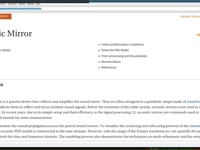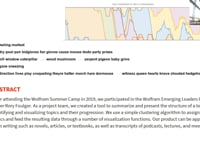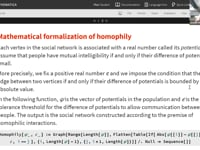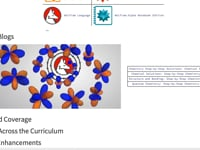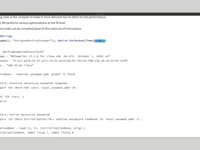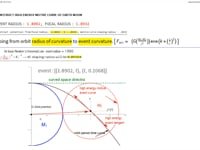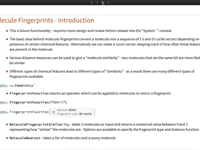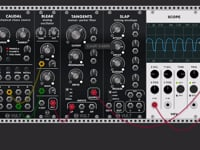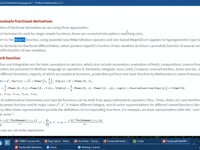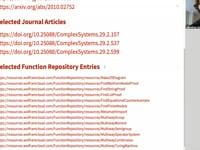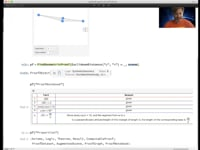Learn how »

# Bonding Like Period Element Atoms Using Parametric Geometry and Z#

In this talk, Alexander Garron discusses the quantum-level parametric geometry he uses to construct and bond like atoms together. ​ ​​ ​Constructing a bonding profile of nuclear energy curves structuring, two like atoms are built with two parametric geometry sections. One section will be atom1 and the other section will be atom2. Let atom1 be south of atom2 and both atoms be separated by a bond plane. Spin axis bond comprising two atoms involves conserved symmetry. Fold any two like atoms along the spin axis, letting east meet west, or fold on the bond plane of rotation, letting north meet south, and profile symmetry of same element nuclear curves will be conserved. Only profile geometry will change to accommodate increasing atomic "weight" by utilizing Z# as an electron cloud radius to construct period elements. Parametric unity geometry is used to construct atom1. A unit circle, ±slope one tangent and tangent normal constructed at +2 latus rectum endpoint of ecloud-dependent curve parabola and nuclear shaping square hyperbola asymptotes are all used to parameterize constructing a nuclear standard model. Protium, hydrogen ((^1)H), is the primitive source standard model I use to derive construction protocol of all period elements. Constructions differ by using element Z# for radius of ecloud. Parametric geometry lines and curves used to construct atom1 are extrapolated to construct atom2. The resulting parametric construction of the two-atom bond will be used to explain the role electromagnetism plays in strengthening the bond of nuclear fields.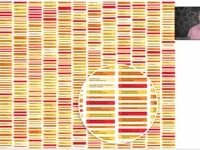The Wolfram Compiler61:14